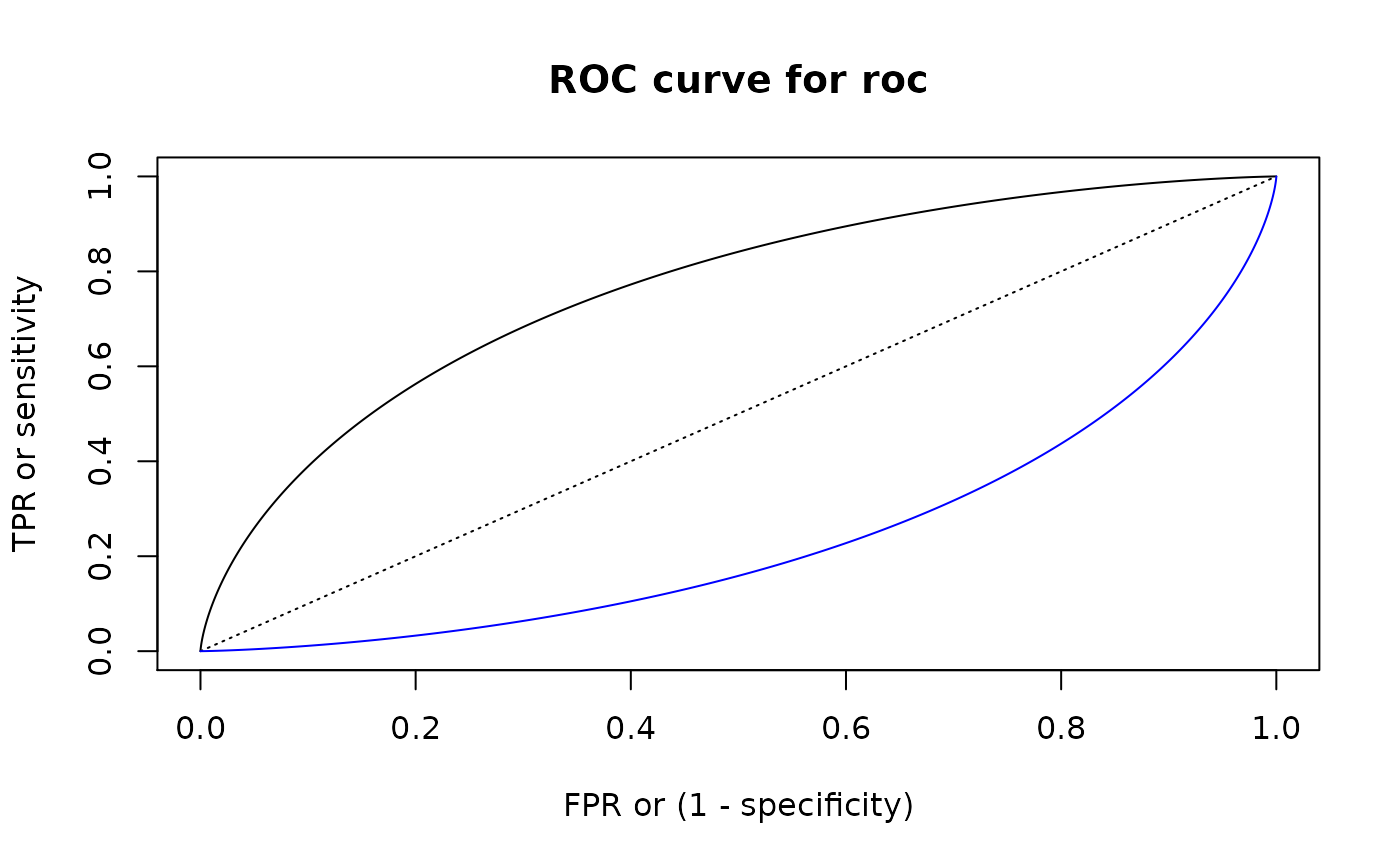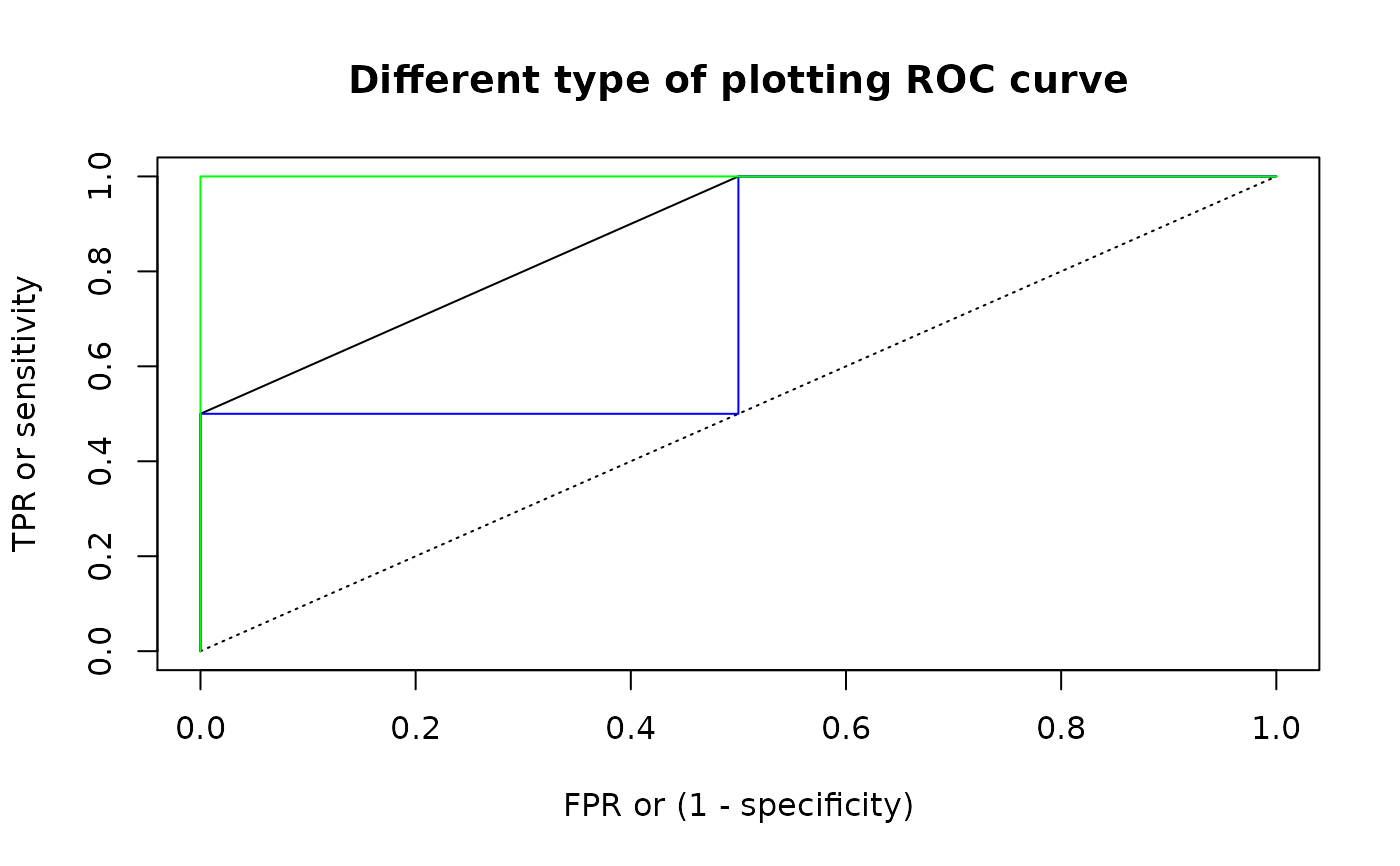These functions help you perform a ROC ("Receiver Operating Characteristic") analysis for one-dimensional linear classifier: values not more than some threshold are classified as "negative", and more than threshold - as "positive". Here input pair of pdqr-functions represent "true" distributions of values with "negative" (f) and "positive" (g) labels.

summ_roc(f, g, n_grid = 1001)

summ_rocauc(f, g, method = "expected")

roc_lines(roc, ...)

## Arguments

f A pdqr-function of any type and class. Represents "true" distribution of "negative" values. A pdqr-function of any type and class. Represents "true" distribution of "positive" values. Number of points of ROC curve to be computed. Method of computing ROC AUC. Should be one of "expected", "pessimistic", "optimistic" (see Details). A data frame representing ROC curve. Typically an output of summ_roc(). Other arguments to be passed to plot() or lines(). If TRUE (default), roc_plot() adds bisector line as reference for "random guess" classifier.

## Value

summ_roc() returns a data frame with n_grid rows and columns "threshold" (grid of classification thresholds, ordered decreasingly), "fpr", and "tpr" (corresponding false and true positive rates, ordered non-decreasingly by "fpr").

summ_rocauc() returns single number representing area under the ROC curve.

roc_plot() and roc_lines() create plotting side effects.

## Details

ROC curve describes how well classifier performs under different thresholds. For all possible thresholds two classification metrics are computed which later form x and y coordinates of a curve:

• False positive rate (FPR): proportion of "negative" distribution which was (incorrectly) classified as "positive". This is the same as one minus "specificity" (proportion of "negative" values classified as "negative").

• True positive rate (TPR): proportion of "positive" distribution which was (correctly) classified as "positive". This is also called "sensitivity".

summ_roc() creates a uniform grid of decreasing n_grid values (so that output points of ROC curve are ordered from left to right) covering range of all meaningful thresholds. This range is computed as slightly extended range of f and g supports (extension is needed to achieve extreme values of "fpr" in presence of "discrete" type). Then FPR and TPR are computed for every threshold.

summ_rocauc() computes a common general (without any particular threshold in mind) diagnostic value of classifier, area under ROC curve ("ROC AUC" or "AUROC"). Numerically it is equal to a probability of random variable with distribution g being strictly greater than f plus possible correction for functions being equal, with multiple ways to account for it. Method "pessimistic" doesn't add correction, "expected" adds half of probability of f and g being equal (which is default), "optimistic" adds full probability. Note that this means that correction might be done only if both input pdqr-functions have "discrete" type. See pdqr methods for "Ops" group generic family for more details on comparing functions.

roc_plot() and roc_lines() perform plotting (with plot()) and adding (with lines()) ROC curves respectively.

summ_separation() for computing optimal separation threshold.

Other summary functions: summ_center(), summ_classmetric(), summ_distance(), summ_entropy(), summ_hdr(), summ_interval(), summ_moment(), summ_order(), summ_prob_true(), summ_pval(), summ_quantile(), summ_separation(), summ_spread()

## Examples

d_norm_1 <- as_d(dnorm)
d_norm_2 <- as_d(dnorm, mean = 1)
roc <- summ_roc(d_norm_1, d_norm_2)
#>   threshold fpr          tpr
#> 1  5.753425   0 0.000000e+00
#> 2  5.742918   0 5.330559e-08
#> 3  5.732412   0 1.093400e-07
#> 4  5.721905   0 1.682311e-07
#> 5  5.711398   0 2.301175e-07
#> 6  5.700891   0 2.951445e-07
# summ_rocauc() is equivalent to probability of g > f
summ_rocauc(d_norm_1, d_norm_2)
#>  0.760251summ_prob_true(d_norm_2 > d_norm_1)
#>  0.760251
# Plotting
roc_plot(roc)
roc_lines(summ_roc(d_norm_2, d_norm_1), col = "blue")# For "discrete" functions summ_rocauc() can produce different outputs
d_dis_1 <- new_d(1:2, "discrete")
d_dis_2 <- new_d(2:3, "discrete")
summ_rocauc(d_dis_1, d_dis_2)
#>  0.875summ_rocauc(d_dis_1, d_dis_2, method = "pessimistic")
#>  0.75summ_rocauc(d_dis_1, d_dis_2, method = "optimistic")
#>  1## These methods correspond to different ways of plotting ROC curves
roc <- summ_roc(d_dis_1, d_dis_2)
## Default line plot for "expected" method
roc_plot(roc, main = "Different type of plotting ROC curve")
## Method "pessimistic"
roc_lines(roc, type = "s", col = "blue")
## Method "optimistic"
roc_lines(roc, type = "S", col = "green")